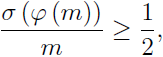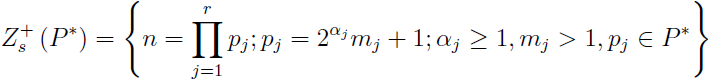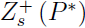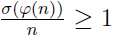# On the composition of the functions σ and φ on the set Zs+(P*)

Aleksander Grytczuk
Notes on Number Theory and Discrete Mathematics, ISSN 1310-5132
Volume 19, 2013, Number 1, Pages 14—18

## Details

### Authors and affiliations

Aleksander GrytczukFaculty of Mathematics, Computer Science and Econometrics
University of Zielona Góra
4a Prof. Szafrana Str., 65-516 Zielona Góra, Poland

### Abstract

In 1964, A. Mąkowski and A. Schinzel (, Cf.) conjectured that for all positive integers m, we have(*)
where σ denote the sum of divisors function and φ is the Euler’s totient function.
Let P be the set of all odd primes and
P* = {pP; p = 2αk + 1; α ≥ 1; k > 1; (k,2) = 1}.
Moreover, letwhere (mj, mk) = 1; for all jk, j, k = 1, 2, …, r. In this paper we prove that if nthen we have. From this and Sándor’s result it follows that (*) is true for all positive integers m ≥ 1 such that the squarefree part of m.

### Keywords

• Arithmetical functions
• Mąkowski—Schinzel conjecture

• 11A25
• 11N37

### References

1. Balakrishnan, U. Some remark on σ(φ(n)), Fibonacci Quarterly, Vol. 32, 1994, 293–296.
2. Cohen, G.L. On a conjecture of Mąkowski and Schinzel, Colloq. Math., Vol. 74, 1997, 1–8.
3. Filaseta, M., S. W. Graham, C. Nicol, On the composition of σ(n) and φ(n), Abstracts AMS, Vol. 13, 1992, No.4, 137.
4. Ford, K. An explicit sieve bound and small values of σ(φ(n)), Period. Math. Hung, Vol. 43, 2001, 15–29.
5. Grytczuk, A., F. Luca, M. Wójtowicz, On a conjecture of Mąkowski and Schinzel concerning the composition of the arithmetic functions σ and φ, Colloq. Math., Vol. 86, 2000, 31–36.
6. Guy, R. K. Unsolved Problems in Number Theory, Springer-Verlag, 1994
7. Luca, F., C. Pomerance, On some problems of Mąkowski and Erdos concerning the arithmetic functions φ and σ, Colloq. Math., Vol. 92, 2002, 111–130.
8. Mąkowski. A., A. Schinzel, On the functions φ (n) and σ(n), Colloq. Math., Vol. 13, 1964/65, 95–99.
9. Redmond, D. Number Theory, Marcel Dekker, Inc., New York, 1996.
10. Sándor, J. On the composition of some arithmetic functions, Studia Babes-Bolyai University, Mathematica, Vol. 34, 1989, 7–14.
11. Sándor, J. Remarks on the functions σ(n) and φ(n), Babes-Bolyai Univ. Seminar on Math. Analysis, Vol. 7, 1989, 7–12.
12. Sándor, J. A note on the functions σ(n) and φ(n), Studia Babes-Bolyai University, Mathematica, Vol. 35, 1990, 3–6.
13. Sándor, J., B. Crstici, Handbook of Number Theory, II, Kluwer Academic Publishers, Dordrecht / Boston / London, 2004.
14. Sándor, J. On the composition of some arithmetic functions, II. J. Inequal. Pure and Appl. Math., Vol. 6, 2005, No. 3, Art. 73.

## Cite this paper

APA

Grytczuk, A. (2013). On the composition of the functions σ and φ on the set Zs+(P*), Notes on Number Theory and Discrete Mathematics, 19(1), 14-18.

Chicago

Grytczuk, Aleksander. “On the Composition of the Functions σ and φ on the Set Zs+(P*).” Notes on Number Theory and Discrete Mathematics 19, no. 1 (2013): 14-18.

MLA

Grytczuk, Aleksander. “On the Composition of the Functions σ and φ on the Set Zs+(P*).” Notes on Number Theory and Discrete Mathematics 19.1 (2013): 14-18. Print.